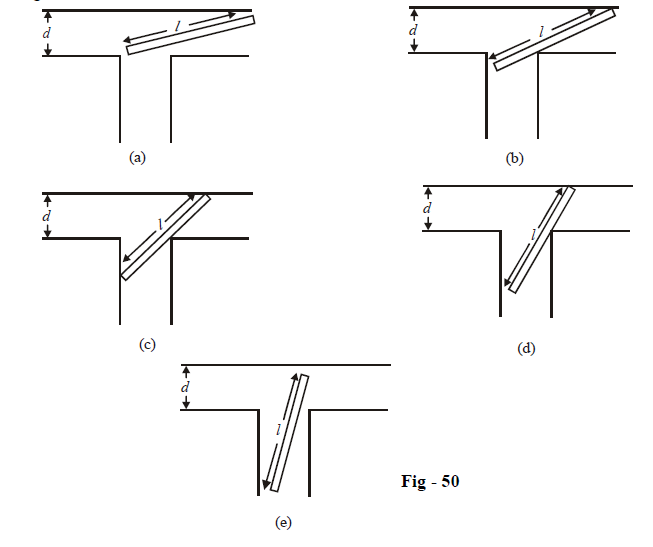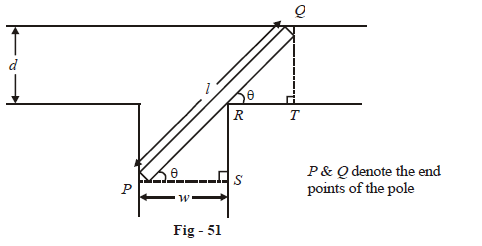# Applications of Derivatives Set 9

Go back to  'SOLVED EXAMPLES'

Example - 19

If f(x) is a twice differentiable function such that f(a) = 0,$$f\left( b \right) = 2,\,\,f\left( c \right) = - 1,\,\,f\left( d \right) = 2$$ ,   $$f\left( e \right) = 0$$  where $$a < b < c < d < e,$$ then find the minimum number of zeroes of $$g\left( x \right)={{\left\{ f'\left( x \right) \right\}}^{2}}+f'\left( x \right)f''\left( x \right)$$  in the interval [a, e]

Solution: Notice that g(x) can be written simply as:

$g\left( x \right) = \frac{d}{{dx}}\left\{ {f'\left( x \right)f\left( x \right)} \right\}$

We let $$f\left( x \right)f'\left( x \right)$$ be represented by h(x).

Therefore,

$g\left( x \right) = \frac{d}{{dx}}\left\{ {h\left( x \right)} \right\}$

Suppose that h(x) has n roots. Let rk and rk+1 represented two successive roots of h(x). For the interval $$\left[ {{r_k},{r_{k + 1}}} \right]$$,  we can apply Rolle’s theorem on h(x) so that:

There exists $$c \in [{r_k},\,\,{r_{k + 1}}]$$  for which $$h'\left( c \right) = 0$$

$$\Rightarrow \quad$$ A zero of h'(x) will lie in $$[{r_k},{r_{k + 1}}]$$

$$\Rightarrow \quad$$ A zero of h'(x) will lie between successive zeroes of h(x)

{This is intuitively obvious}

$$\Rightarrow \quad h'\left( x \right)$$ has n–1 zeroes

Now we first find the number of roots of $$h\left( x \right) = 0$$ or$$f\left( x \right)f'\left( x \right) = 0$$

$\,\text{i}\text{.e}\,\,f\left( x \right)=0\,\,\text{or}\,\,f'\left( x \right)=0$

From the given data on f(x), it must have at least 4 zeroes (at a, between b and c, between c and d and at e)

$$\Rightarrow \quad f'\left( x \right)$$ must have at least 3 zeroes (one  between every pair of successive     zeroes of (x))

$$\Rightarrow \quad f\left( x \right)f'\left( x \right)$$ or h (x) must have minimum 7 zeroes

$$\Rightarrow \quad h'\left( x \right)$$ must have minimum 6 zeroes

Example - 20

If $$a,b,c \in \mathbb{R}\,\,{\text{and}}\,\,a + b + c = 0,$$  show that the equation $$3a{x^2} + 2bx + c = 0$$has at least one root in [0, 1]

Solution: Notice that the expression $$3a{x^2} + 2bx + c$$  can be obtained from the differentiation of $$f\left( x \right) = a{x^3} + b{x^2} + cx + d$$where d is an arbitrary constant.

We will now try to use f(x) to prove the stated assertion. Since the question mentions the interval [0, 1], we should first find out f(0) and f(1):

$\begin{array}{l}f\left( 0 \right) = d\\f\left( 1 \right) = a + b + c + d = d\,\,\,\,\,\,\,\,\,\,\,\,\,\,\,\,\,\,\,\,\,\,\,\,\,\,\,\,\,\,\,\,\,\,\,\,\,\,\,\,\left( {{\rm{since}}\,\,\,a + b + c = 0} \right)\end{array}$

Also, since f(x) is a polynomial function, it is differentiable and hence Rolle’s theorem can be applied to it for the interval [0, 1]:

There exists at least one $$p \in [0,\,\,1]$$ such that $$f'\left( p \right) = 0$$

i.e $$3a{x^2} + 2bx + c = 0$$ for at least one $$p \in [0,\,1]$$

$$\Rightarrow \,3a{x^2} + 2bx + c = 0$$ has at least one root in [0, 1]

Example - 21

A pole $$l$$ feet long is to be carried horizontally from one corridor to another corridor perpendicular to each other. If the first corridor is ‘d’ meters wide, find the minimum required width of the other corridor.

Solution: The situation described in the question is depicted in the sequence figure below, as the pole is being moved from one corridor to the other.Let the width of the second corridor be w. Convince yourself that if w is below a particular value (if the second corridor is too narrow), the pole will never be able to be moved into the second corridor.

Now, consider the case when w has a value such that the pole is just able to move into the second corridor. This situation is depicted in the figure above. Observe (and visualise in your mind) carefully that an instant will come when the ends of the pole touch the walls of both the corridors and the pole touches the corner (turning). This is depicted in Fig - 50 part (c) and reproduced below in more detail:For the entire movement of the rod from one corridor to the other (while all the time touching the point R), the instant depicted above will be the one when the horizontal component of PR, i.e PS, will be maximum. Before and after this instant, PS will have a lower value. Therefore, the width w must be at least greater than the maximum value of PS; only then will the pole pass:

$${w_{\min }} = P{S_{\max }}$$

Therefore, our aim is to find PSmax.

From the geometry of the figure, we can write:

\begin{align}&\,\,\,\,\,\,\,\,\,\,\,\,\,\,\,\,\,\,d\,{\rm{cosec}}\theta + \left( {PS} \right)\sec \theta = l\\\\ &\Rightarrow \quad PS = \frac{{\ell - d\,{\rm{cosec}}\,\theta }}{{\sec \theta }} = \ell \cos \theta - d\cot \theta \end{align}

For maximum PS,       \begin{align}\frac{{d\left( {PS} \right)}}{{d\theta }} = 0\,\,{\rm{and}}\,\,\frac{{{d^2}\left( {PS} \right)}}{{d{\theta ^2}}} < 0\end{align}

\begin{align}\frac{{d\left( {PS} \right)}}{{d\theta }} = - \ell \sin \theta + d\,{\rm{cose}}{{\rm{c}}^2}\theta \end{align}

This is 0 when

\begin{align}\;\ell \sin \theta = d\,{\rm{cose}}{{\rm{c}}^2}\theta \\\\ \Rightarrow \quad \sin \theta = {\left( {\frac{d}{\ell }} \right)^{1/3}}\\\end{align}

Verify that \begin{align}{\left. {\frac{{{d^2}\left( {PS} \right)}}{{d{\theta ^2}}}} \right|_{\theta = {{\sin }^{ - 1}}{{\left( {\frac{d}{\ell }} \right)}^{1/3}}}}\end{align}  < 0

\begin{align}&\Rightarrow \quad\; \sin \theta\;\; = {\left( {\frac{a}{\ell }} \right)^{1/3}}\text{ is a point of maximum for PS}\\\\&{\left. { \Rightarrow \quad P{S_{\max }} = \ell \cos \theta - d\cot \theta } \right|_{\theta = {{\sin }^{ - 1}}{{\left( {\frac{d}{\ell }} \right)}^{1/3}}}}\\\\& \qquad\qquad\quad= {\left( {{\ell ^{2/3}} - {d^{2/3}}} \right)^{3/2}}\\\\ &\Rightarrow \;\quad {w_{\min }} \;= {\left( {{\ell ^{2/3}} - {d^{2/3}}} \right)^{3/2}}\end{align}

Learn from the best math teachers and top your exams

• Live one on one classroom and doubt clearing
• Practice worksheets in and after class for conceptual clarity
• Personalized curriculum to keep up with school